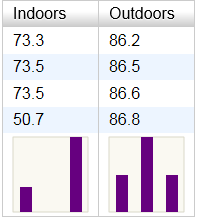## Wednesday, June 26, 2013

### Create empty DataFrame in Pandas

It seems like it should be a simple thing: create an empty DataFrame in the Pandas Python Data Analysis Library. But if you want to create a DataFrame that

• is empty (has no records)
• has datatypes
• has columns in a specific order

...i.e. the equivalent of SQL's CREATE TABLE, then it's not obvious how to do it in Pandas, and I wasn't able to find any one web page that laid it all out. The trick is to use an empty Numpy ndarray in the DataFrame constructor:

```df=DataFrame(np.zeros(0,dtype=[
('ProductID', 'i4'),
('ProductName', 'a50')]))
```

Then, to insert a single record:

```df = df.append({'ProductID':1234, 'ProductName':'Widget'})
```

UPDATE 2013-07-18: Append is missing a parameter:

`df = df.append({'ProductID':1234, 'ProductName':'Widget'},ignore_index=True)`

## Tuesday, June 18, 2013

### Histogram thumbnails inside YUI3 data grids

Sparklines are tiny graphs added inline to data tables or lists. Sparklines have no units or gridlines; the purpose is just to quickly convey the shape of the data, which is usually presented adjacent.

A powerful variant on sparklines are sparkline histograms, or "sparkgrams" as I like to call them. Sparkgrams can quickly and compactly convey data distributions: outliers, right/left skew, normal vs. uniform distribution, etc. Because they are small, they can be included right inside a data table for every column or row. Such a presentation is useful either for completely known data when embarking upon data analysis, or for newly received data of a known data type in, for example, a manufacturing quality control scenario.

Below is an example of how it might look. The first column demonstrates an outlier, while the second column conveys a normal distribution.Below is the YUI3 code to render the above.

```<!DOCTYPE html>
<script src="yui/build/yui/yui-min.js"></script>
<script>
YUI().use('datatable', 'charts', function(Y) {
var fmt = function(o) {
if (o.value.substring && o.value.substring(0,5) === "chart")
o.cell.set("innerHTML", "<div id='" + o.value +
"' style='width:75px;height:75px'></div>");
else
o.cell.set("text", o.value);
return false;
}

var table = new Y.DataTable({columns: [{"key":"Indoors",  "nodeFormatter":fmt},
{"key":"Outdoors", "nodeFormatter":fmt}],
data: [{"Indoors": 73.3, "Outdoors": 86.2},
{"Indoors": 73.5, "Outdoors": 86.5},
{"Indoors": 73.5, "Outdoors": 86.6},
{"Indoors": 50.7, "Outdoors": 86.8},
{"Indoors": "chart0", "Outdoors": "chart1"}],
render: "#table"});

for (col = 0; col < table._displayColumns.length; col++) {
var a = Array();
for (row = 0; row < table.get("data").size()-1; row++)
a.push(table.getCell([row,col]).get("text"));
var NUM_BINS = 3;
var amin = Math.min.apply(Math, a);
var binwidth = 1.001 * (Math.max.apply(Math, a)-amin) / NUM_BINS;
var data=Array();
for (i=0; i<NUM_BINS; i++)
data.push({category:amin+i*binwidth, values:0});
for (i=0; i<a.length; i++)
data[Math.floor((a[i]-amin)/binwidth)].values++;
new Y.Chart({dataProvider: data, type:"column",
axes: {values: {position:"none"}, category: {position:"none"}},
render:"#chart"+col});
}
});
</script>
<body>
<div id="table" class="yui3-skin-sam"></div>
</body>
</html>
```

## Thursday, June 13, 2013

### Query Hive from iPython Notebook

iPython Notebook together with pandas comprise a data analysis system that is essentially a clone of R. The advantage over R is that Python code can be more easily converted into production code and executed, for example, on a web server.

One limitation is that the Apache-recommended Python interface to Hive requires installing Hive locally, which can be problematic or inconvenient from, say, a laptop used for analysis. A nice alternative is remotely executing Hive on the Hadoop cluster (or adjacent Linux server with access to the cluster). The alternative does require installing sshpass. At the end of the code below, the query results are stored in a pandas DataFrame with column names and automatic detection of data types.

```from pandas import *
from StringIO import StringIO

s = "sshpass -f myfilewithpassword ssh myusername@myhostname \"hive -S -e \\\"" \
"SELECT * from mytable;\\\"\""

t = !\$s
```

UPDATE 2013-08-01: It's a bit cleaner if one uses Python's triple-quoting mechanism as below. This allows one to copy and paste queries between iPython Notebook and Hive and Hue without having to reformat with quotes and backslashes.

```from pandas import *
from StringIO import StringIO

s = """